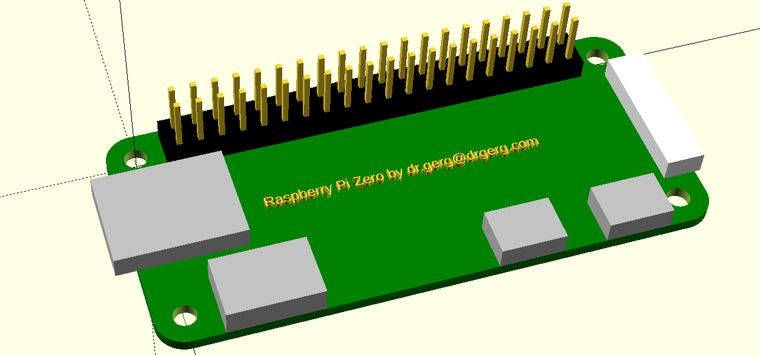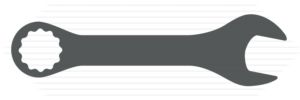When I build things using the Prusa 3D printer, I like to also create a model of the part I'm making brackets or enclosures for. That way I can see how the whole thing is going to fit together without making bad assumptions about how any particular part is going to fit in the overall assembly.Of course, the point of the exercise is to produce a model that has the important pieces that need holes or space on the interior of an enclosure. You have to make sure you account for such things, and this way is a great way to do that. Like the SD card sticking out the left. It's the whole card, not just the holder. And the camera connector on the other end also sticks out .68mm from the PCB edge. It could be important someday, you never know.

So, without further ado, here are the two text files that create this model. First is the 40-pin header followed by the Pi which uses the 40-pin header file:

``````//Dr.Gerg's 40-pin 2.54mm Header
// https://www.drgerg.com
// PARAMETER DEFINITIONS
module pin(tx,ty,tz,ry,cl,cx,cy){
translate([tx,ty,tz])
rotate([0,ry,0])
cube([cl,cx,cy],false);
translate([-2,-1,3])
color("black")
cube([51.4,5.23,2.54],false);
}
translate([2,.9875,0])
for (i=[0:19])
pin(i*2.54,0,0,270,12,.64,.64);
translate([2,.9875,0])
for (i=[0:19])
pin(i*2.54,2.54,0,270,12,.64,.64);
}
``````
``````//Dr.Gerg's Raspberry Pi Zero Model
// https://www.drgerg.com
// PARAMETER DEFINITIONS

\$fn=48;
difference(){
color("green")
minkowski(){
cube([23.1,58.1,.7]);
cylinder(h=.7,r=3.45,center=false);
}
cylinder(h=6,d=2.7,center=true);
translate([30-6.9,0,0])
cylinder(h=6,d=2.7,center=true);
translate([30-6.9,65-6.9,0])
cylinder(h=6,d=2.7,center=true);
translate([0,65-6.9,0])
cylinder(h=6,d=2.7,center=true);
}
translate([5.23/2,(58.1/2)-(51.4/2),-1.4])
rotate([0,0,90])
color("silver")
translate([23.1+3.45-7.63,3.73,1.4])
cube([7.63,11.21,3.25]);
color("silver")
translate([23.1+3.45-5.68,47.79,1.4])
cube([5.68,7.68,2.71]);
color("silver")
translate([23.1+3.45-5.68,35,1.4])
cube([5.68,7.68,2.71]);
color("silver")
translate([3.83,-(3.45+2.56),1.4])
cube([12,15.38,2.71]);
color("white")
translate([23.1/2-17.05/2,58.1+3.45-(4.5-.68),1.4])
cube([17.05,4.5,2.71]);
translate([23.1/2,58.1/2,1])
rotate([0,0,90])
linear_extrude(1)
text("Raspberry Pi Zero by dr.gerg@drgerg.com",1.3,halign="center");
``````